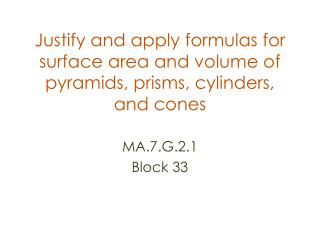DownloadDownload PresentationJustify and apply formulas for surface area and volume of pyramids, prisms, cylinders, and cones

# Justify and apply formulas for surface area and volume of pyramids, prisms, cylinders, and cones

Download Presentation## Justify and apply formulas for surface area and volume of pyramids, prisms, cylinders, and cones

- - - - - - - - - - - - - - - - - - - - - - - - - - - E N D - - - - - - - - - - - - - - - - - - - - - - - - - - -
##### Presentation Transcript

1. Justify and apply formulas for surface area and volume of pyramids, prisms, cylinders, and cones MA.7.G.2.1 Block 33

2. Learning Outcomes • Understanding of Volume • Find Surface Areas and Volumes for Prisms, Cylinders, Pyramids, Cones, and Spheres. • Formulate possible formulas for volume for the 3-dimensional figures mentioned before Teacher Quality Grant

3. Vocabulary needed height, pyramid, height, cross- section sphere, great circle, cylinder, radius, right cylinder, oblique cylinder, altitude, cone • polyhedron, • edge, • vertex, • tetrahedron, • regular polyhedron, • bases, • lateral faces • lateral edges, • prism, • oblique prism, • altitude, Teacher Quality Grant

4. GeoGebra files needed • Rectangular_prism.zip • Right_triangular_prism.zip • Triangular_prism.zip • Rectangula_pyramid.zip • Triangular_pyramid.zip • Cone_worksheets.ggb • Cylinder_worksheet.ggb • Sphere.zip Teacher Quality Grant

5. Procedure • Discuss the formula used to find the volume of a prism. Talk about what the "B" means in relation to the formula: area of the base, which is a rectangle. Discuss the dimensions needed in order to calculate volume: length and width of base and height of prism. Teacher Quality Grant

6. Look at the GeoGebra File for a Rectangular Prism. Calculate the volume and surface area Changes to dimensions can be done easily by manipulating the sliders to create hundreds of examples. Formulas and solutions can be shown or hidden on the worksheets. • Experiment how the volume and surface area change as you proportionately change the dimensions, using both an enlargement and a reduction. Is there a relationship to scale factor? If so, what is it? • The same steps can be used for the rest of the files Teacher Quality Grant

7. National Library of Virtual Manipulatives • On the web, go to: • http://nlvm.usu.edu • Click on the square: • 6-8 grade, Geometry • Select the applet: How High • http://nlvm.usu.edu/en/nav/frames_asid_275_g_3_t_3.html?from=category_g_3_t_3.html Teacher Quality Grant

8. How would you solve these problems? Teacher Quality Grant